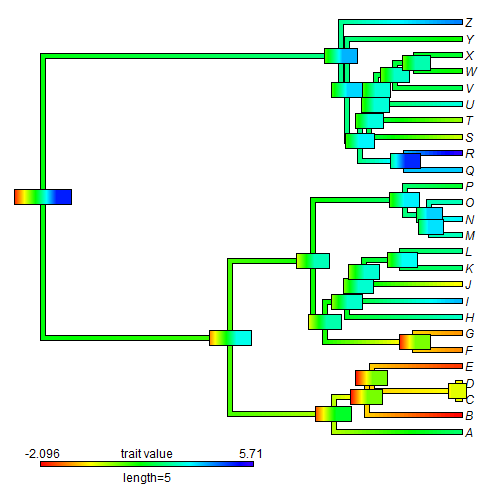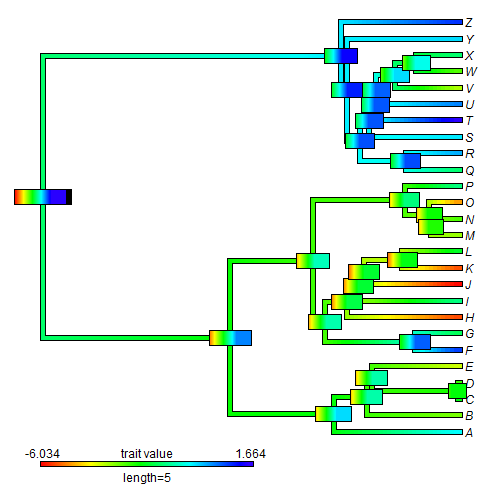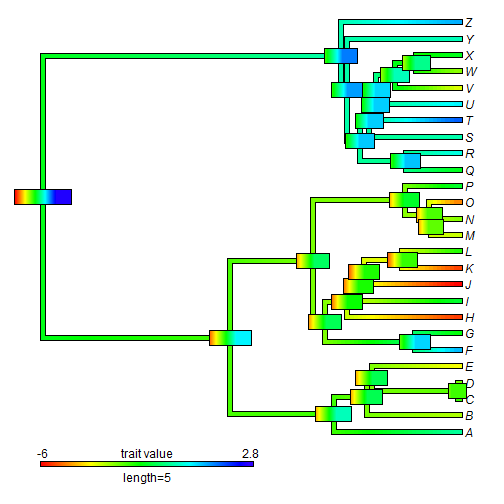## Monday, February 27, 2017

### Function to add error bars to a `contMap` plot

I just wrote a function to add error bars to a `"contMap"` plot, as discussed today on R-sig-phylo and my blog.

The function looks as follows:

``````errorbar.contMap<-function(obj,...){
if(hasArg(x)) x<-list(...)\$x
else x<-setNames(sapply(1:Ntip(obj\$tree),function(x,obj){
ii<-which(obj\$tree\$edge[,2]==x)
ss<-names(obj\$tree\$maps[[ii]][length(obj\$tree\$maps[[ii]])])
obj\$lims+as.numeric(ss)/(length(obj\$cols)-1)*diff(obj\$lims)
},obj=obj),obj\$tree\$tip.label)
if(hasArg(scale.by.ci)) scale.by.ci<-list(...)\$scale.by.ci
else scale.by.ci<-FALSE
tree<-obj\$tree
aa<-fastAnc(tree,x,CI=TRUE)
xlim<-range(aa\$CI95)
if(xlim>obj\$lims||xlim<obj\$lims){
cat(paste("  -----\n  The range of the contMap object, presently (",
round(obj\$lims,4),",",
round(obj\$lims,4),
"), should be equal to\n  or greater than the range of the CIs on ancestral states: (",
round(xlim,4),",",round(xlim,4),").\n",sep=""))
cat(paste("  To ensure that your error bars are correctly plotted, please recompute your\n",
"  contMap object and increase lims.\n  -----\n",sep=""))
}
d<-diff(obj\$lims)
if(scale.by.ci){
v<-aa\$CI95[,2]-aa\$CI95[,1]
v<-v/max(v)
} else v<-rep(0.5,tree\$Nnode)
n<-length(obj\$cols)-1
lastPP<-get("last_plot.phylo",envir=.PlotPhyloEnv)
h<-max(nodeHeights(tree))
for(i in 1:tree\$Nnode){
ii<-round((aa\$CI95[i,1]-obj\$lims)/d*n)
jj<-round((aa\$CI95[i,2]-obj\$lims)/d*(n+1))
cols<-obj\$cols[ii:jj]
x=lastPP\$xx[i+Ntip(tree)]-0.05*h*v[i],
y=lastPP\$yy[i+Ntip(tree)],title="",subtitle="",lims=NULL,
lwd=14)
}
}
``````

One element of the function

``````x<-setNames(sapply(1:Ntip(obj\$tree),function(x,obj){
ii<-which(obj\$tree\$edge[,2]==x)
ss<-names(obj\$tree\$maps[[ii]][length(obj\$tree\$maps[[ii]])])
obj\$lims+as.numeric(ss)/(length(obj\$cols)-1)*diff(obj\$lims)
},obj=obj),obj\$tree\$tip.label)
``````

is a complicated piece of code that is only used if the optional argument `x` (the same as `x` in `contMap` is not supplied. What this line does is pull the values of `x` instead from the mapped object.

OK, let's try it:

``````library(phytools)
tree
``````
``````##
## Phylogenetic tree with 26 tips and 25 internal nodes.
##
## Tip labels:
##  A, B, C, D, E, F, ...
##
## Rooted; includes branch lengths.
``````
``````x
``````
``````##           A           B           C           D           E           F
##  2.40577324 -2.09590799 -0.03881523 -0.32857686 -1.71533031 -1.07523748
##           G           H           I           J           K           L
## -0.99890853  3.07436198  4.00842163 -0.27299915  2.41135389  2.61494639
##           M           N           O           P           Q           R
##  2.97710788  3.56872630  2.96244691  1.92234400  3.85672218  5.70984852
##           S           T           U           V           W           X
##  0.15119803  0.50825014  3.26386662  2.21301397  1.52734360  1.93244087
##           Y           Z
##  1.51311417  4.41672993
``````
``````y
``````
``````##           A           B           C           D           E           F
## -0.52075890 -3.22896754 -1.99922219 -2.99064026 -3.85137945  0.92981725
##           G           H           I           J           K           L
## -1.77583525 -5.53973459 -1.39568877 -6.03400572 -5.50545828 -2.04112670
##           M           N           O           P           Q           R
## -3.45201728 -2.87475079 -4.97170923 -1.41198149 -1.58924448  0.07361102
##           S           T           U           V           W           X
## -0.37121255  1.66386064  0.38763598 -3.65979548 -2.99468975 -1.57039289
##           Y           Z
## -0.27934165  0.97554201
``````

First with `x`:

``````obj<-contMap(tree,x,plot=FALSE)
plot(obj,xlim=c(-0.5,10.5))
errorbar.contMap(obj,scale.by.ci=TRUE)
``````Now with `y`:

``````obj<-contMap(tree,y,plot=FALSE)
plot(obj,xlim=c(-0.5,10.5))
errorbar.contMap(obj,scale.by.ci=TRUE)
````````````##   -----
##   The range of the contMap object, presently (-6.034,1.6639), should be equal to
##   or greater than the range of the CIs on ancestral states: (-5.9555,2.7099).
##   To ensure that your error bars are correctly plotted, please recompute your
##   contMap object and increase lims.
##   -----
``````

This tells us that the range of the CIs on the ancestral nodes for `y` exceeds the limits of the `"contMap"` object. This is why some of the plotted CIs (most noticeably, the root) have black regions. This should be easy to fix as follows:

``````obj<-contMap(tree,y,lims=c(-6,2.8),plot=FALSE)
plot(obj,xlim=c(-0.5,10.5))
errorbar.contMap(obj,scale.by.ci=TRUE)
``````Some of the kinks are still being worked out. Please pardon any bugs!

1.1.